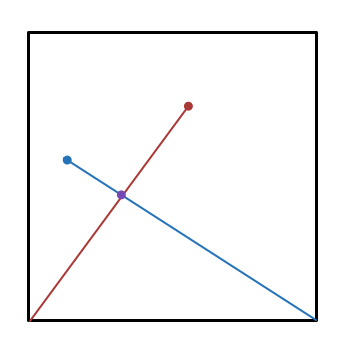# An intersection of geometry and combinatorics

Probability Level 4Consider a square with vertices $(0,0), (0,1), (1,1)$ and $(1,0)$. Choose a random point within the square and draw a line segment from it to $(0,0)$. Next, choose a second random point within the square and draw a line segment from this point to $(1,0)$.

The probability that these two line segments intersect is $\frac{a}{b}$, where $a$ and $b$ are positive coprime integers. Find $a + b$.

×

Problem Loading...

Note Loading...

Set Loading...